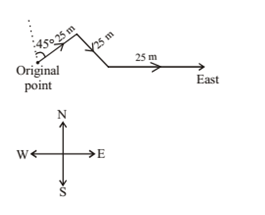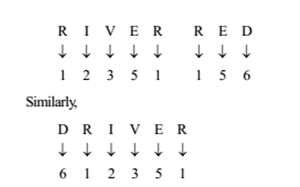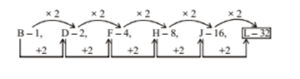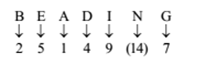# RRB ALP 2018 Practice Test Papers | Reasoning Questions (Day-34)

Dear Aspirants, Here we have given the Important RRB ALP & Technicians Exam 2018 Practice Test Papers. Candidates those who are preparing for RRB ALP 2018 can practice these Reasoning Questions to get more confidence to Crack RRB 2018 Examination.

[WpProQuiz 2052]

Click “Start Quiz” to attend these Questions and view Solutions

1. If “+” means “minus”, “×” means “divided by”, ” ¸ ” means “plus” and “–” means “multiplied by”, then 126 × 14 + 7 – 3 ¸ 2 =?

(a) – 10

(b) – 12

(c) – 17

(d) – 41

1. In the following question, which one set of letters when sequentially placed at the gaps in the given letter series shall complete it? d _ ba_c_a_cb_

(a) cdbda

(c) bdacd

(d) abdca

1. Neeraj is facing north, then he turns 45 degree right and goes 25 m, then turns in south – east direction to move 25 m and from there 25 m to east. In which direction/place is he from his original place?

(a) North

(b) East

(c) West

(d) South

1. Introducing a boy Ankit said, “He is the son of daughter of my grandfather’s son”. How is that boy related to Ankit?

(a) Cousin

(b) Brother

(c) Father – in – law

(d) Nephew

1. Five boys A, B, C, D and E are standing in a line. A is taller than E but shorter than D. B is shorter than E and C is the tallest. Who is in the middle?

(a) A

(b) C

(c) D

(d) E

1. In a certain code language, “RIVER” is written as “12351” and “RED” is written as “156”. How is “DRIVER” written in that code language?

(a) 612311

(b) 612531

(c) 621351

(d) 612351

1. B-1, D-2, F-4, H-8, J-16,?.

(a) K-64

(b) L-32

(c) M-32

(d) L-64

1. If A = 1, B = 2 and N = 14, then BEADING =?

(a) 2154 (14) 97

(b) 2514 (14) 79

(c) 25149 (14) 7

(d) 2154(14)79

1. Among 5 boys, Vasant is taller than Manohar, but not as tall as Raju. Jayant is taller than Dutta but shorter than Manohar. Who is the tallest in the group?

(a) Manohar

(b) Vasant

(c) Jayant

(d) Raju

1. If 73 + 46 = 42 and 95 + 87 = 57, then 62 + 80 =?

(a) 32

(b) 48

(c) 64

(d) 36

126 ÷ 14 – 7 × 3 + 2 = – 10

dcba/ dcba/ dcba

1. Answer: (b)Nephew.

C > D > A > E > B So, A is in the middle.

1. Answer: (d)2. Answer: (b)3. Answer: (c)According to given condition; the correct order is: Raju>Vasant>Manohar>Jayant>Dutta \ Raju is the tallest among them.

As, 73 + 46 = 42

7 – 3 = 4, 4 + 6 = 10

4 + 10 = 14

14 × 3 = 42

Similarly, 6 – 2 = 4, 8 + 0 = 8

4 + 8 = 12

12 × 3 = 36

RRB ALP 2018 Practice Test Papers | Reasoning Questions (Day-1)

RRB ALP 2018 Practice Test Papers | Reasoning Questions (Day-2)

RRB ALP 2018 Practice Test Papers | Reasoning Questions (Day-3)

RRB ALP 2018 Practice Test Papers | Reasoning Questions (Day-4)

RRB ALP 2018 Practice Test Papers | Reasoning Questions (Day-5)

RRB ALP 2018 Practice Test Papers | Reasoning Questions (Day-6)

RRB ALP 2018 Practice Test Papers | Reasoning Questions (Day-7)

RRB ALP 2018 Practice Test Papers | Reasoning Questions (Day-8)

RRB ALP 2018 Practice Test Papers | Reasoning Questions (Day-9)

RRB ALP 2018 Practice Test Papers | Reasoning Questions (Day-10)

RRB ALP 2018 Practice Test Papers | Reasoning Questions (Day-11)

RRB ALP 2018 Practice Test Papers | Reasoning Questions (Day-12)

RRB ALP 2018 Practice Test Papers | Reasoning Questions (Day-13)

RRB ALP 2018 Practice Test Papers | Reasoning Questions (Day-14)

RRB ALP 2018 Practice Test Papers | Reasoning Questions (Day-15)

RRB ALP 2018 Practice Test Papers | Reasoning Questions (Day-16)

RRB ALP 2018 Practice Test Papers | Reasoning Questions (Day-17)

RRB ALP 2018 Practice Test Papers | Reasoning Questions (Day-18)

RRB ALP 2018 Practice Test Papers | Reasoning Questions (Day-19)

RRB ALP 2018 Practice Test Papers | Reasoning Questions (Day-20)

RRB ALP 2018 Practice Test Papers | Reasoning Questions (Day-21)

RRB ALP 2018 Practice Test Papers | Reasoning Questions (Day-22)

RRB ALP 2018 Practice Test Papers | Reasoning Questions (Day-23)

RRB ALP 2018 Practice Test Papers | Reasoning Questions (Day-24)

RRB ALP 2018 Practice Test Papers | Reasoning Questions (Day-25)

RRB ALP 2018 Practice Test Papers | Reasoning Questions (Day-26)

RRB ALP 2018 Practice Test Papers | Reasoning Questions (Day-27)

RRB ALP 2018 Practice Test Papers | Reasoning Questions (Day-28)

RRB ALP 2018 Practice Test Papers | Reasoning Questions (Day-29)

RRB ALP 2018 Practice Test Papers | Reasoning Questions (Day-30)

RRB ALP 2018 Practice Test Papers | Reasoning Questions (Day-31)

RRB ALP 2018 Practice Test Papers | Reasoning Questions (Day-32)

RRB ALP 2018 Practice Test Papers | Reasoning Questions (Day-33)